## Example Questions

### Example Question #1 : How To Find A Solution To A Compound Fraction

4/5 + 7/3 + 9/30 = ?

80 / 30

20 / 38

103 / 30

20 / 30

30 / 100

103 / 30

Explanation:

To add fractions you must first find the lease common denominator, that is, a number that all of the denominators (the bottom number) can divide into.

In this case, 5, 3, and 30, both 5 and 3 divide successfully into 30, so 30 is the least common denominator.

Now divide each smaller denominator into thirty.  Then multiple the answer by the top number:

5/30 = 6

Therefore 4/5 = 4*6 / 5*6 = 24/30

3/30 = 10

Therefore 7*10 / 3*10 = 70/30

Then add all the numerators together:

24/30 + 70/30 +9/30 = (24+70+9)/30 = 103 / 30

### Example Question #2 : How To Find A Solution To A Compound Fraction

The student body of a high school is 1/4 boys, and 2/3 of the boys are seniors. If 1/18 of the senior boys were accepted into Harvard, which of the following could be the total number of students in the class?

108

90

15

54

148

108

Explanation:

For this problem it would be easier to choose one of the answers and go from there. 108 works because 1/4 of 108 is 27 then 2/3 of 27 is 18 and 1/18 of 18 is 1, which makes this answer possible.

The other answers come out with a remainders that make no sense, since a fractional part of a person cannot have been accepted to a school.

### Example Question #3 : How To Find A Solution To A Compound Fraction

Compute: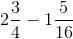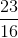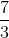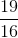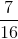Explanation:

Rewrite each fraction into an improper fraction.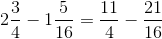The common denominator is 16.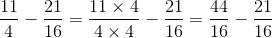The correct answer is: